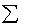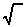previous lesson

Eta

ASSOCIATION: one nominal, one interval variable

A. Eta n: ungrouped data:

n2 = {[nj (Ymj - Ym)2 ] } / {Y2-- [(Y)2 / N]}

Where: Y = score of interval variable
Ymj = mean for nominal category
Ym = mean for total sample
nj = number in a nominal category
N = total in sample
k = number of nominal categories

Example: Ungrouped data

 Union income Non-union income 10 2 10 3 5 2 5 3

Q: What is the association between union membership and income?
A:

 Y=union (u) Y2u Y=non-union (nu) Y2nu 10 100 2 4 10 100 3 9 5 25 2 4 5 25 3 9 30 = Yu 10 = Ynu nu= 4 nnu= 4

nu = 4 ----nnu = 4

Yu = 30/4 = 7.50 -----Ynu = 10/4 = 2.50

Total Sample: Y = Yu + Ynu = 40

N = nu + nnu = 8

Y = Y/N = 40/8 = 5.0

Y2 = 276

---Union category -----non-union category
n2 ={[4(7.50 - 5.00)2 ] + [4 (2.50 - 5.00)2]}/{276 - [(40)2/8]}

n2 = .66

n =.66 = .31

Interpretation:

: Use scale "There is ______association between (variable 1) and (variable 2)."Interpretation for n2: Convert to a percent and include in the statement, "_____% of the variance in (interval variable) can be explained by (nominal variable)." or 1 - n2: Convert to a percent and include in the statement, "_____% of the variance in (interval variable) cannot be explained by (nominal variable)."

Example: .31 = a moderately small association between income and union membership.

n2 = .66: "66% of variance in income can be explained by union membership."

1 - n2 = .34: "34% of variance in income cannot be explained by union membership."

B. Eta--grouped data

n2 = {[nj(Ymj - Ym)2 ]} / {f(Y)2 -- [(fY)2 / N]}

Where: f = frequency
Y = score of interval variable
Ymj = mean for nominal category
Ym = mean for total sample
nj = number in a nominal category
N = total in sample
k = number of nominal categories

Hint: Ym =fm/N; Yj =fm/nj

Example: grouped data

 Income Class '69 Class '71 Class '82 f f f 10 2 0 0 5 1 1 5 4 0 1 0 2 0 2 0

Q: What is the association between graduating class and income?
A:

 Income Class '69 Class '71 Class '82 f fm f fm f fm 10 2 20 0 0 0 0 5 1 5 1 5 5 25 4 0 0 1 4 0 0 2 0 0 2 4 0 0 3= n69 25 2= n71 13 5= n82 25

Ym69 = 25/3 = 8.33; Ym71 = 13/4 = 3.25; Ym82 = 25/5 = 5.00

Total Sample:

 Income f fm or Y Y2 fY2 10 2 20 100 200 5 7 35 25 175 4 1 4 16 16 2 2 4 4 8 12 = N 63 399

Ymtotal =fm/N = 63/12 = 5.25

n2 = [3(8.33 - 5.25)2] + [4(3.25 - 5.25)2] + [5(5.00 - 5.25)2 ]

399 - [(63)2 /12]

n2 = .66

n= .81

Interpret these the same as for Eta for ungrouped data.